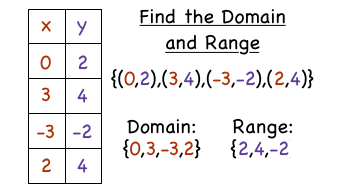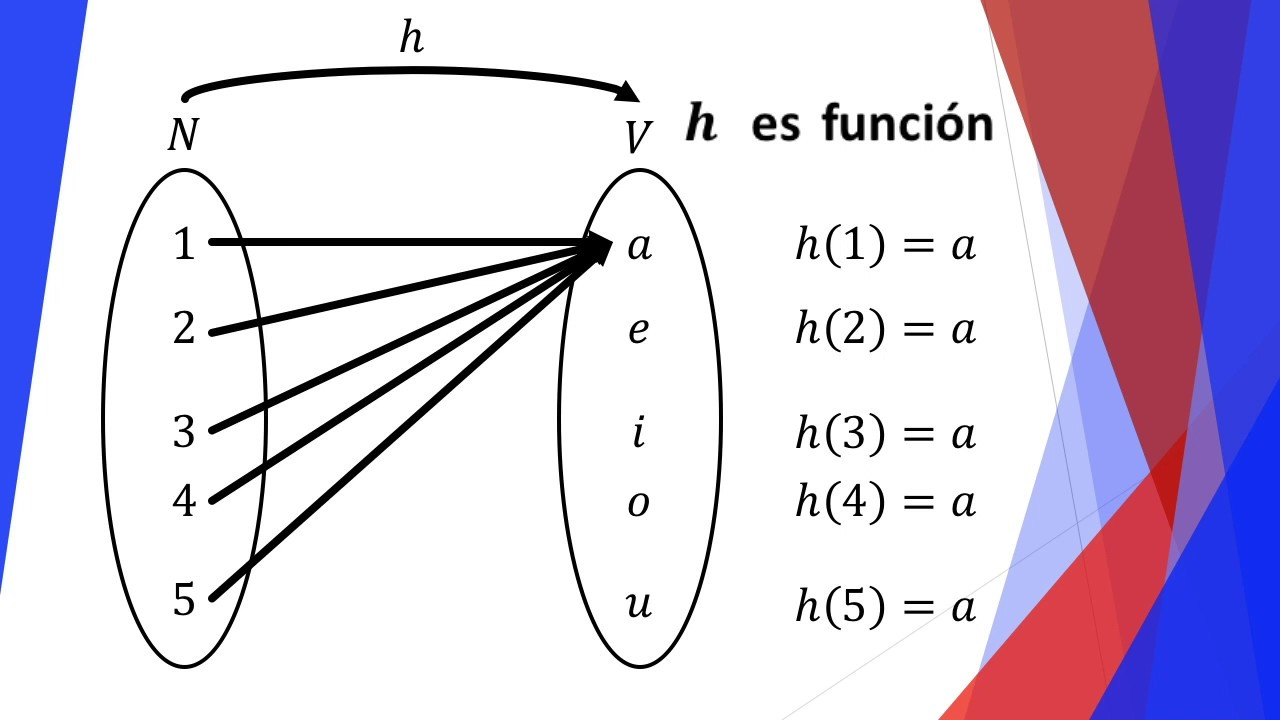# Waves Lab

Transverse wave: Wave which oscillations are perpendicular to the direction of the wave

Longitudinal wave: The oscillations are parallel to the direction of the wave

Periodic wave: Have a repeating continuous pattern that determines its  wavelength

Pulse wave: a waveform that includes squares waves and similarly periodic but asymmetric waves

# week#13

Slope-intercept form

We use this formula: y=mx+b

where m is the slope and b is the y intercept

example:

m=3

b=2

y=3x+2

# week #12

Slope: Tells you the direction of a line and you can calculate it with the following formula:

m: y2-y1/x2-x1

m: slope# Thermos Challenge

• Planning, process, and Analysis:
1. Prototype testing procedure:1. Thermos prototype organizers and images:
1. Temperature/time graph• Final Product and results:(Materials include: 1 styrofoam cup, aluminum foil, tape)

• Conclusion:

The design sacrifices its aesthetic appeals to the low cost of building the thermos prototype (at \$2.03). The isolated design as a whole is to have as fewer holes between the foil cover and the coffee cup as possible, to minimalize the heat loss due to water turning into other forms (e.g., steam). An insulative container was used (as in this prototype, a styrofoam coffee cup) to minimalize heat loss due to conduction (since the cup conducts heat slower compared to other materials, at only 0.03 W/m•K). It also has just the right volume to contain 150 ml of water to slow down the heat loss of the water due to the convection (the small space of air on top of it). The aluminum foil’s more reflective side is used to reflect radiation caused by the body of hot water.

After 45 minutes of the experiment with 150 ml of water (80° at the time of starting), we found out that the total heat loss was kept under 38° (28° at the time of recording), which is meeting our initial expectations.

Overall, the design’s objective is to prioritize maximizing heat-keeping capabilities over every other factor.

# Week #11: Middle Point

Middle point: Is the point that divides a line in 2 equal parts and it can be determined by the following formula:

P(x,y)=(x1+x2)/2 , (y1+y2)/2

# Week post #9

Domain: It is in the independent variable (x) and it can be explained as all the possible x values that are gonna make the function work and will output real y values

Range: It is in the dependent variable (y) and are all the resulting variables of the y values after we substituting all the x values# Week #10: Functions

A function is a relation between the independent and the dependent variable, where the independent variable receives only 1 partner of the dependent. If the independent receives 2 partners is not a function but the dependents can receive 2 or more.